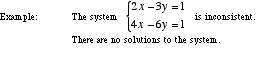index: click on a letter A B C D E F G H I J K L M N O P Q R S T U V W X Y Z A to Z index index: subject areas numbers & symbols sets, logic, proofs geometry algebra trigonometry advanced algebra & pre-calculus calculus advanced topics probability & statistics real world applications multimedia entrieswww.mathwords.com about mathwords website feedback

 Inconsistent System of Equations A system of equations which has no solutions. Note: Attempts to solve inconsistent systems typically result in impossible statements such as 0 = 3.See also